## 10.12.14

### Generate a Fibonacci series between 0 and 1000. The first two initial value are 0 and 1

#include<iostream>
using namespace std;
class fibonacci
{
public:
void set_digit (int m, int n)
{
f1=m;
f2=n;
}
protected:
int f1;
int f2;
int f3;
};

class print : public fibonacci
{
public:
void show_print()
{
int maxnum;
cout <<"Enter the maximum number of fibonacci series: "<< "\n";
cout <<"\nPrinting fibonacci series from 0 to 1000: " << "\n");
while (f1+f2 < = 1000)
{
f3=f1+f2;
cout <<f3 <<"\n";
f1=f2;
f2=f3;
}
}
};
int main()
{
print p;
p.set_digit (0, 1);
p.show_print( );
}

#### 16 comments:

1.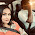This comment has been removed by the author.

1.Why have you deleted? Submit your code part by part. or You may share your file using dropbox.

2.This comment has been removed by the author.

3.This comment has been removed by the author.

4.This comment has been removed by the author.

5.#include
using namespace std;

class fibonacci
{
public:
void setnum(int a, int b)
{
n1=a;
n2=b;

}
protected:
int n1;
int n2;
int n3;
};

class series:public fibonacci
{
public:
void get_series()
{
cout << "The Fibonacci Series iss : is: \n";
while (n1+n2<1000)
{
n3 = n1 + n2;
n1 = n2;
n2 = n3;
cout << n3 << " ";
}

}
};

int main()
{
series s;
s.setnum(0,1);
s.get_series();
}

1.noted and found correct

6.This comment has been removed by the author.

7.#include
using namespace std;
//base class
class fibo
{
public:
void setget(int n)
{
get=n;
}
protected:
int get;
};
//derived class
class number:public fibo
{
public:
int f1=0,f2=1,f3,n;
int code()
{
while(1)
{
f3=f1+f2;
if(f1>n)
break;
cout<<f3<<" ";
f1=f2;
f2=f3;
}
}
};

8.int main()
{
number nb;
int a;
cin>>a;
nb.setget(a) ;
nb.code();
}

9.due to publishing problem i couldnt posted my full program code here,so i divided it into two parts...and posted 1st half 1st,2nd half below

10.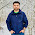#include
using namespace std;
class fibonacci
{
public:
void set_digit(int m,int n)
{

f1=m;
f2=n;

}

protected:
int f1;
int f2;
int f3;
};

class print:public fibonacci
{
public:
void show_print()
{
int n;
cout<<"Enter the maximum number of fibonacci series: "<>n;
cout<<"\nPrinting fibonacci series from 0 to 1000: "<n)

break;
cout<<f3<<"\n";
f1=f2;
f2=f3;

}
}
};
int main()
{
print p;
p.set_digit(0,1);
p.show_print();
}

11.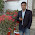//Generate fibonacci series using inheritance
//Md. Imam Uddin
//ID: 201420733

#include
#include

using namespace std;
class fibonacci
{
public:
void num(int a,int b)
{
n1=a;
n2=b;
}
protected:
int n1;
int n2;
int n3;
};
class s:public fibonacci
{
public:
void get_series()
{
cout << "The fibonacci series is: ";
while(n1+n2<100)
{
n3=n1+n2;
n1=n2;
n2=n3;
cout<<n3<<" ";
}
}
};
int main()
{
s p;
p.num(0, 1);
p.get_series();
getche();

}

12.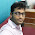Md : jalis Mahmud
Id : 201420762

#include
using namespace std;
class fibonacci
{
public:
void set_digit(int a,int b)
{

m1=a;
m2=b;

}

protected:
int m1;
int m2;
int m3;
};

class print:public fibonacci
{
public:
void show_print()
{
int n;
cout<<"Enter the maximum number of fibonacci series: "<>b;
cout<<"\nPrinting fibonacci series from 0 to 500: "<b)

break;
cout<<f3<<"\b";
f1=m2;
f2=m3;

}
}
};
int main()
{
print p;
p.set_digit(0,1);
p.show_print();
}

13.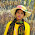#include
using namespace std;
class fibonacci
{
public:
void set_digit(int a, int b)
{
d1=a;
d2=b;
}
protected:
int d1;
int d2;
int d3;
};

class series:public fibonacci
{
public:
void get_series()
{
cout << "The Fibonacci Series is: \n";
while (d1+d2<1000)
{
d3 = d1 + d2;
d1 = d2;
d2 = d3;
cout << d3 << " ";
}

}
};

int main()
{
series r;
r.set_digit(0,1);
r.get_series();
}

14.#include
using namespace std;

class p
{
public:
int n,l;
int m;
void input()
{

cin>>n>>l;
}

void output()
{

while(l+n<1000)
{
m=n+l;
n=l;
l=m;
cout<<m<<" ";
}

}
};
int main()
{
p s;
s.input();
s.output();
return 0;
}

Comment Here